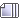###Author Topic: The one unforgivable sin is to be boring  (Read 715 times)

0 Members and 0 Guests are viewing this topic.

#### mic check##### an aside: sum of decimal fractions as sum of geometric progression
« Reply #15 on: February 01, 2020, 09:43:49 pm »
UPDATE:  all inlcuded in one PDF file if that is more convenient:
(see this post)

______________________________________________________________

0.55 ... = 5/9

How?   The second 5 is repeating, so this is an infinite geometric series.

0.55 ... = 0.5 + 0.05 + 0.005 + ...

first term is 1/2, and the ratio 0.05/0.5 = 5/50 = 1/10, so the sum of the terms of this infinite geometric progression (sequence), called an infinite geometric series [the Sum, that is the series], would be (1/2)/(1-1/10) = (1/2)/(9/10) = (1/2)*(10/9) = 5/9

The notes included allow the spontaneous use of the vinculum over the repeating digits, but here on this board I would be forced to use bold or something to indicate such.    I have to run.   I hope the notes give you some insight into the numbers system's relation to infinite series ....

just a prelude to the Binomial Theorem notes.   Maybe printing hard copy with hand-written notes, printed from device to actual hard copy print might trigger a sense of self-respect and dignity in yourself to validate your quest for understanding.

Prelude to Binomial Theorem ... 1 ...

prelude page 2

____________________________________________________________
TESTING 1, 2 ...

(will direct to another thread with door to jump into next post in this thread)

« Last Edit: February 02, 2020, 05:11:28 pm by mwh »
Things They Will Never Tell YouArthur Schopenhauer has been the most radical and defiant of all troublemakers.

Gorticide @ Nothing that is so, is so DOT edu

~ Tabak und Kaffee Süchtigen ~